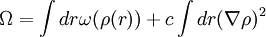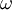# Van der Waals' density gradient theory$\Omega = \int dr \omega(\rho(r)) + c \int dr (\nabla\rho)^2$,
where a local approximation is employed in the first term ($\omega$ being the grand potential density of the bulk system), and the variation in the density profile enters in the second term. This second term is the integral of the square of the density gradient, with a proportionality constant that is termed the influence parameter.